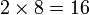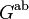# Second cohomology group for trivial group action of Q8 on Z2

This article gives information about the second cohomology group for trivial group action (i.e., the second cohomology group with trivial action) of the group quaternion group on cyclic group:Z2. The elements of this classify the group extensions with cyclic group:Z2 in the center and quaternion group the corresponding quotient group. Specifically, these are precisely the central extensions with the given base group and acting group.
The value of this cohomology group is Klein four-group.
Get more specific information about quaternion group |Get more specific information about cyclic group:Z2|View other constructions whose value is Klein four-group

## Description of the group

We consider here the second cohomology group for trivial group action of the quaternion group on cyclic group:Z2, i.e.,$H^2(G,A)$

where$G \cong Q_8$ and$A \cong \mathbb{Z}_2$.

The cohomology group is isomorphic to Klein four-group.

## Elements

FACTS TO CHECK AGAINST (second cohomology group for trivial group action):
Background reading on relationship with extension groups: Group extension problem
Arithmetic functions of extension group:
order (thus all extension groups have the same order): order of extension group is product of order of normal subgroup and quotient group
nilpotency class: nilpotency class of extension group is between nilpotency class of quotient group and one more for central extension
derived length: derived length of extension group is bounded by sum of derived length of normal subgroup and quotient group
minimum size of generating set: minimum size of generating set of extension group is bounded by sum of minimum size of generating set of normal subgroup and quotient group|minimum size of generating set of quotient group is at most minimum size of generating set of group
WHAT'S THE TABLE BELOW?: Recall that there is a correspondence:
Elements of the group$H^2(G;A)$ for the trivial group action$\leftrightarrow$ congruence classes of central extensions with the specified subgroup$A$ and quotient group$G$.
This descends to a correspondence:
Orbits for the group action of$\operatorname{Aut}(G) \times \operatorname{Aut}(A)$ on$H^2(G;A)$$\leftrightarrow$ pseudo-congruence classes of central extensions.
The table below breaks down the second cohomology group as a union of these orbits, with (as a general rule) each row describing one orbit, i.e., one "cohomology class type", aka one "pseudo-congruence class" of central extensions. The number of rows is the number of pseudo-congruence classes of central extensions.

Note that all these extensions are central extensions with the base normal subgroup isomorphic to cyclic group:Z2 and the quotient group isomorphic to quaternion group. Due to the fact that order of extension group is product of order of normal subgroup and quotient group, the order of each of the extension groups is$2 \times 8 = 16$.

None of the extensions are stem extensions, because the Schur multiplier of the quaternion group is a trivial group. (In general, stem extensions are possible only if the base of the stem extension is a quotient of the Schur multiplier).

Cohomology class type Number of cohomology classes Corresponding group extension GAP ID (second part, order is 16) Stem extension? Base characteristic in whole group? Hall-Senior family (equivalence class up to being isoclinic) Nilpotency class of whole group Derived length of whole group Subgroup information on base in whole group
trivial 1 direct product of Q8 and Z2 12 No No$\Gamma_2$ 2 2 PLACEHOLDER FOR INFORMATION TO BE FILLED IN: [SHOW MORE]
nontrivial 3 nontrivial semidirect product of Z4 and Z4 4 No Yes$\Gamma_2$ 2 2 central subgroup generated by a non-square in nontrivial semidirect product of Z4 and Z4
Total (--) 4 -- -- -- -- -- -- -- --

## Group actions

### Under the action of the automorphism group of the quaternion group

The automorphism group of the quaternion group is isomorphic to symmetric group:S4, with the inner automorphisms sitting as a normal Klein four-subgroup inside that, and the quotient isomorphic to symmetric group:S3. This automorphism group acts on$H^2(G,A)$ by pre-composition, and under this action, the automorphism group is transitive on the three nontrivial cohomology classes. In fact, it acts as symmetric group:S3 on them, with the kernel being the normal Klein four-subgroup given by the inner automorphisms.

## Direct sum decomposition

For background information, see formula for second cohomology group for trivial group action in terms of second homology group and abelianization

### General background

We know from the general theory that there is a natural short exact sequence:$0 \to \operatorname{Ext}^1_{\mathbb{Z}}(G^{\operatorname{ab}},A) \to H^2(G;A) \stackrel{\operatorname{Skew}}{\to} \operatorname{Hom}(H_2(G;\mathbb{Z}),A) \to 0$

where$G^{\operatorname{ab}}$ is the abelianization of$G$ and its image comprises those extensions where the restricted extension of the derived subgroup$[G,G]$ on$A$ is trivial and the corresponding extension of the quotient group is abelian. We also know, again from the general theory, that the short exact sequence above splits, i.e., the image of$\operatorname{Ext}^1_{\mathbb{Z}}(G^{\operatorname{ab}},A)$ in$H^2(G;A)$ has a complement inside$H^2(G;A)$. However, there need not in general be a natural or even an automorphism-invariant choice of splitting.

### In this case

For this choice of$G$ and$A$,$G^{\operatorname{ab}}$ is a Klein four-group, hence$\operatorname{Ext}^1(G^{\operatorname{ab}},A)$ is also a Klein four-group. Further, the Schur multiplier$H_2(G;\mathbb{Z})$ is the trivial group, hence$\operatorname{Hom}(H_2(G;\mathbb{Z}),A)$ is also trivial. Thus, the image of$\operatorname{Ext}^1$ is the whole of$H^2$. The direct sum decomposition of$H^2$ that we obtain therefore is a trivial decomposition in the sense that one of the direct summands is the whole group.

## GAP implementation

### Construction of the cohomology group

The cohomology group can be constucted using the GAP functions SmallGroup, TwoCohomology, TrivialGModule, GF.

gap> G := SmallGroup(8,4);;
gap> A := TrivialGModule(G,GF(2));;
gap> T := TwoCohomology(G,A);
rec( group := <pc group of size 8 with 3 generators>, module := rec( field := GF(2), isMTXModule := true, dimension := 1,
generators := [ <an immutable 1x1 matrix over GF2>, <an immutable 1x1 matrix over GF2>, <an immutable 1x1 matrix over GF2> ] ),
collector := rec( relators := [ [ [ 3, 1 ] ], [ [ 2, 1, 3, 1 ], [ 3, 1 ] ], [ [ 3, 1 ], [ 3, 1 ], 0 ] ], orders := [ 2, 2, 2 ],
wstack := [ [ 3, 1 ], [ 2, 1, 3, 1 ], [ 3, 1 ] ], estack := [  ], pstack := [ 3, 5, 3 ], cstack := [ 1, 1, 1 ], mstack := [ 0, 0, 0 ],
list := [ 1, 0, 1 ], module := [ <an immutable 1x1 matrix over GF2>, <an immutable 1x1 matrix over GF2>, <an immutable 1x1 matrix over GF2> ],
mone := <an immutable 1x1 matrix over GF2>, mzero := <an immutable 1x1 matrix over GF2>, avoid := [  ], unavoidable := [ 1, 2, 3, 4, 5, 6 ] ),
cohom := <linear mapping by matrix, <vector space of dimension 3 over GF(2)> -> ( GF(2)^2 )>,
presentation := rec( group := <free group on the generators [ f1, f2, f3 ]>,
relators := [ f1^2*f3^-1, f1^-1*f2*f1*f3^-1*f2^-1, f2^2*f3^-1, f1^-1*f3*f1*f3^-1, f2^-1*f3*f2*f3^-1, f3^2 ] ) )

### Construction of extensions

The extensions can be constructed using the additional command Extensions.

gap> G := SmallGroup(8,4);;
gap> A := TrivialGModule(G,GF(2));;
gap> L := Extensions(G,A);;
gap> List(L,IdGroup);
[ [ 16, 12 ], [ 16, 4 ], [ 16, 4 ], [ 16, 4 ] ]

### Under the action of the various automorphism groups

This uses additionally the GAP functions AutomorphismGroup, DirectProduct, CompatiblePairs, and ExtensionRepresentatives.

gap> G := SmallGroup(8,4);;
gap> A := TrivialGModule(G,GF(2));;
gap> A1 := AutomorphismGroup(G);;
gap> A2 := GL(1,2);;
gap> D := DirectProduct(A1,A2);;
gap> P := CompatiblePairs(G,A,D);;
gap> M := ExtensionRepresentatives(G,A,P);;
gap> List(M,IdGroup);
[ [ 16, 12 ], [ 16, 4 ] ]